Area and perimeter of overlap of two circles

strantor

Joined Oct 3, 2010
5,752
Not homework help, this is a real world application. I have a large "knife gate" valve used at the bottom of a hopper full of granular product. I need to know the hydraulic diameter (4x cross-sectional area / perimeter) in order to calculate the flow through it for a given opening width. The orifice of the knife gate is a 16" circle, and the flow is cut by a plate with a semicircular edge with a radius of 10.25"In the picture above, the cross-hatched area is the area through which product can flow, if the knife gate is open to a width of 1.125"
I need to know cross-sectional area and perimeter (arc length a + arc length b) of the cross-hatched area.
Currently I am getting this data from AutoCAD. AutoCAD tells me the arc lengths and the area of the cross-hatch, so I move the knife in AutoCAD 1/8" at a time and record the arc lengths and area into a look-up table for a piece-wise linear scaling operation.This is very tedious. I will continue the drudgery but I would much prefer a formulaic solution.

I have googled all of the things, and the results I get are much more complicated than I want them to be, and are exclusively solving for the area of two circles like this: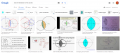Not the crescent shape I need. And also not giving arc lengths/perimeter.

Is there any better way than what I'm already doing?

•cmartinez

Papabravo

Joined Feb 24, 2006
17,605
Fortuneately the arc length of a circle is known in closed form. You need to know the radii of the two circles and the angle subtended by the the shaded inntersection. Arc length is just:

$$s\;=\;(r_1+r_2)\theta$$

•strantor

Tesla23

Joined May 10, 2009
495
From: Wikipedia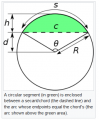The area shaded green is given by: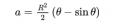You have the difference in areas of two circular segments with the same common chord 'c', you know the two radii, you just have to work out the two angles θ which you can do by straightforward trig.

•strantor

strantor

Joined Oct 3, 2010
5,752
Thanks, I have made good progress. I am now properly calculating the angles from the center of both circles to the point of intersection of the circles, and the length of the chord, that is far enough for today.

This doesn't seem to work though:
Simple example: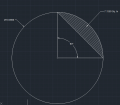I tried both radians and degrees: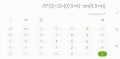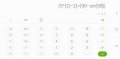Last edited:

Tesla23

Joined May 10, 2009
495
•strantor

strantor

Joined Oct 3, 2010
5,752
Thanks I don't know why I wasn't able to make the equation work. I tried on the phone calculator you see in the screenshot, in Excel, on the Windows calculator, and in my PLC. All gave the same result. I found that equation on multiple websites, so it must be correct and I'm just entering the data incorrectly. Whatever, I'm past it. I ended up just calculating the area of the sector, then calculating the area of the triangle formed by the chord, and subtracting the triangle.

Papabravo

Joined Feb 24, 2006
17,605
Most calculators have three settings for angle measure. They are
1. Radians - 2π for the whole circle
2. Degrees - 360 ° for the whole circle
3. Grads - 400 for the whole circle
If I set my calculator to grads I get your first reault of 19.327
It kinda looks lile you might have ignored that detail. Check it out

•atferrari and strantor

strantor

Joined Oct 3, 2010
5,752
This is now resolved. I am successfully simulating flow rates through a crescent-shaped orifice thanks to you guys' help. In the highly unlikely case that you're as curious as I was about the significance of entering equivalent circular diameter [SQRT(cross-sectional area/pi)*2] vs hydraulic diameter [4*cross-sectional area/perimeter] into the Beverloo equation for bulk solids flow rate through an orifice, the answer is that it is very significant.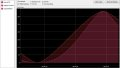Next step is to see how the theoretical stacks up against the empirical.

•cmartinez

MrAl

Joined Jun 17, 2014
8,616
Most problems involving 2d geometry can be solved by simply solving the equation(s) that describe the object(s). Intersection problems are really just equating two or more curves and finding the solution(s).
Simple example:
e1=x+1
e2=2*x
The solution is found by equating the two:
e1=e2
which is:
x+1-2*x
and solving for x we get x=1.

Circles are no difference except there may be more than one intersection point and so you get more than one solution, but that's often what you want anyway. That provides you with the limits needed to find areas also so you can integrate from one limit to the next.
From there you can generalize into simpler formulas and/or procedures.
You can look up the equations for circles in the plane and that will provide you with the starting point.
For example:
R^2=(x-h)^2+(y-k)^2
where h and k are the center x and y coordinates of the center of the circle. If you have two circles then you have two equations:
Ra^2=(x-h1)^2+(y-k1)^2
Rb^2=(x-h2)^2+(y-k2)^2
and so solve these you can solve for y in each and then equate the two results.. There is always the possibility you may have to find the solution numerically.
I'll provide more details if needed.

•strantor

MrChips

Joined Oct 2, 2009
25,040The area shaded green is given by:Area of a circle is
$$a = \pi r^2$$

Hence $$(\theta - sin \theta)$$ must be in radians.

For example, if $$\theta = \frac{\pi}{2}$$ , i.e. a quarter of a circle,

$$a = \frac{r^2}{2}(\theta - sin \theta)$$

$$a = \frac{r^2}{2}(\frac{\pi}{2} - sin (\frac{\pi}{2}))$$

which works out to be the same as:

$$a = \frac{\pi r^2}{4} - \frac{r^2}{2}$$

which is a quarter circle minus the triangle.

•strantor

strantor

Joined Oct 3, 2010
5,752
Nothing to do with circles... if you came for circles, I'm sorry.

In case anyone was actually interested, or more likely, for anyone who comes across this thread after googling Beverloo, or other bulk solids flow related terms, below are my findings.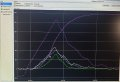The purple line that goes up and settles at 1500 is the weight dispensed through the gate. The other purple line is the knife position ×100 (peaks around 13.5 inches open). Blue is the expected fill rate from the Beverloo equation using equivalent circular diameter of the real-time orifice dimensions. Green is the expected fill rate from the Beverloo equation using hydraulic diameter of the real-time orifice dimensions. White is the actual fill rate derived from rate of change from the load cell, so it's not an instantaneous value to start, plus it's mildly averaged because real-time is too jumpy to make any sense of. Despite that, I think the linear slope of the white line fits the equivalent circle diameter equation better than the hydraulic diameter equation.

If you disagree with my conclusion based on something you see just from looking at this one screenshot (I know, it might seem like it could go either way), know that I've watched the chart populate hundreds of times running dozens of different products throug the orifice and have a mental library of these screenshots that I'm basing my conclusion on. IMO There's no point in calculating perimeter and/or hydraulic diameter if you're dealing with a similar application.

•cmartinez